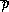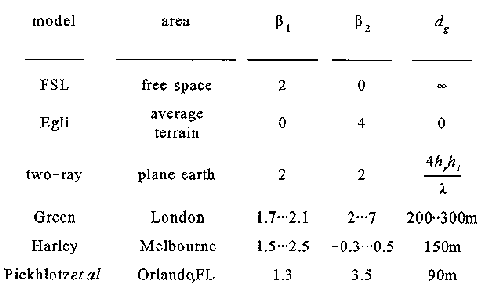### JPL's Wireless Communication Reference Website

#### Chapter: Wireless Channels Section: Path Loss# Micro-Cellular Path LossMicrocellular networks use a cell size of, say, 200 to 2,000 meters. Propagation models for micro-cellular communication typically model the path loss law as a transition from free-space propagation to groun wave propagation if d < dg where theoretically the turnover distance dg occurs at dg < 4hR hT / l, where d is the distance of the radio link under study, hR and hT are the heights of the receiving and transmitting antenna respectively, and l is the wavelength of the transmitted wave.

Various models have been proposed, e.g. a step-wise transition from "20 log d" to "40 log d" at a certain (turnover) distance.

• Harley suggested a smooth transition, withwhere r is a normalized distance, with r = d / R, with d the propagation distance in meters and R the cell size in meters. Similarly rg = dg / R is the normalized turnover distance, andis the local-mean power (i.e., received power averaged over a few meters to remove to effect of multipath fades). Studies indicate that actual turnover distances are on the order of 800 meters around 2 GHz.

• Other models, such as a stepwise transition, have been proposed. Empirical values for the path loss exponents and their intervals of validity have been reported in many papers.A smooth transition is often considered in theoretical system studies.

## Multipath in Micro-Cells

The micro-cellular propagation channel typically is Rician fading: it contains a dominant direct component, with an amplitude determined by path loss, a set of early reflected waves adding (possibly destructively) with the dominant wave, and intersymbol interference caused by the excessively delayed waves, adding incoherently with the dominant wave.